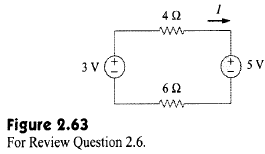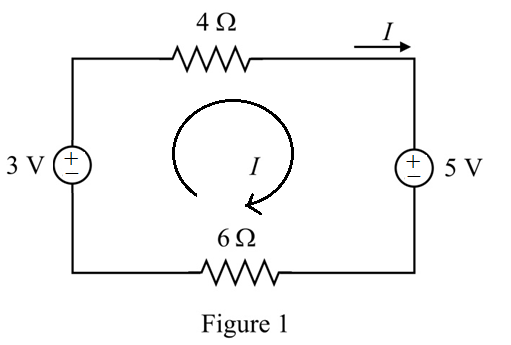Problem

# The current I in the circuit of Fig. 2.63 is:(a) –0.8A(b) –0.2A(c) 0.2A(d) 0.8AAnswer:b

The current I in the circuit of Fig. 2.63 is:

(a) –0.8A

(b) –0.2A

(c) 0.2A

(d) 0.8A#### Step-by-Step Solution

Solution 1

Refer to Figure 2.63 in the textbook.

Draw the modified circuit diagram.Apply Kirchhoff's Voltage Law to the circuit.

\begin{aligned} &-3+4 I+5+6 I=0 \\ &10 I+2=0 \\ &10 I=-2 \\ &I=\frac{-2}{10} \\ &=-0.2 \mathrm{~A} \end{aligned}

The value of loop current is -0.2A.

The correct option is b.